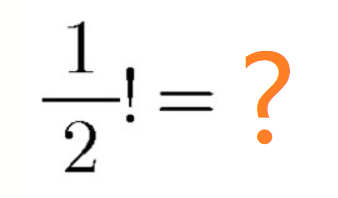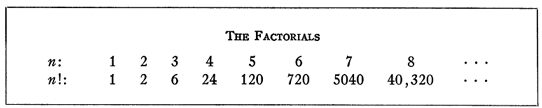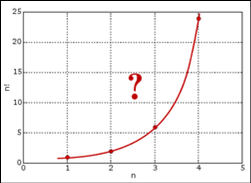【答案】 1728年，哥德巴赫在考虑数列插值的问题时，准备把数列从整数集合延拓到实数集合。例如数列 $1,4,9,16...$ 可以看成函数 $y=n^2$, 这里$n$为整数，但是如果我们把$n$扩展到实数$x$时，$y=x^2$ 仍然成立，这就把把定义在整数集上的公式延拓到实数集合。$$\frac{1 \cdot 2 \cdot 3 \cdots m}{(1+n)(2+n) \cdots(m-1+n)}\left(m+\frac{n}{2}\right)^{n-1} \rightarrow n !$$

$$\left[\left(\frac{2}{1}\right)^n \frac{1}{n+1}\right]\left[\left(\frac{3}{2}\right)^n \frac{2}{n+2}\right]\left[\left(\frac{4}{3}\right)^n \frac{3}{n+3}\right] \cdots=n !$$

$$\lim _{m \rightarrow \infty} \frac{1 \cdot 2 \cdot 3 \cdots m}{(1+n)(2+n) \cdots(m+n)}(m+1)^n=n ! \quad(* *)$$

\begin{aligned} & \frac{1 \cdot 2 \cdot 3 \cdots m}{(1+n)(2+n) \cdots(m+n)}(m+1)^n \\ = & 1 \cdot 2 \cdot 3 \cdots n \cdot \frac{(n+1)(n+2) \cdots m}{(1+n)(2+n) \cdots m} \cdot \frac{(m+1)^n}{(m+1)(m+2) \cdots(m+n)} \\ = & n ! \frac{(m+1)^n}{(m+1)(m+2) \cdots(m+n)} \\ = & n ! \prod_{k=1}^n \frac{m+1}{m+k} \rightarrow n ! \quad(m \rightarrow \infty) \end{aligned}

$$\left(\frac{1}{2}\right) !=\sqrt{\frac{2 \cdot 4}{3 \cdot 3} \cdot \frac{4 \cdot 6}{5 \cdot 5} \cdot \frac{6 \cdot 8}{7 \cdot 7} \cdot \frac{8 \cdot 10}{9 \cdot 9} \cdots}$$

$$\frac{2 \cdot 4}{3 \cdot 3} \cdot \frac{4 \cdot 6}{5 \cdot 5} \cdot \frac{6 \cdot 8}{7 \cdot 7} \cdot \frac{8 \cdot 10}{9 \cdot 9} \cdots=\frac{\pi}{4}$$

$$\left(\frac{1}{2}\right) !=\frac{\sqrt{\pi}}{2}$$

$$J(e, n)=\int_0^1 x^e(1-x)^n d x$$

$$J(e, n)=\frac{n}{e+1} J(e+1, n-1)$$

$$J(e, n)=\frac{1 \cdot 2 \cdots n}{(e+1)(e+2) \cdots(e+n+1)}$$

$$n !=(e+1)(e+2) \cdots(e+n+1) \int_0^1 x^e(1-x)^n d x$$

$$n !=\int_0^1(-\log t)^n d t$$

$$n !=\int_0^{\infty} u^n e^{-u} d u$$

$$\Gamma(x)=\int_0^1(-\log t)^{x-1} d t=\int_0^{\infty} t^{x-1} e^{-t} d t$$

$$\Gamma(n+1)=\int_0^{\infty} \mathrm{e}^{-x} x^{n+1-1} \mathrm{~d} x=\int_0^{\infty} \mathrm{e}^{-x} x^n \mathrm{~d} x$$

$$\int_0^{\infty} \mathrm{e}^{-x} x^n \mathrm{~d} x=\left[\frac{-x^n}{\mathrm{e}^x}\right]_0^{\infty}+n \int_0^{\infty} \mathrm{e}^{-x} x^{n-1} \mathrm{~d} x$$

$$\lim _{x \rightarrow \infty} \frac{-x^n}{\mathrm{e}^x}=\lim _{x \rightarrow \infty} \frac{-n ! \cdot 0}{\mathrm{e}^x}=0 .$$

$$\Gamma(n+1)=n \int_0^{\infty} \frac{x^{n-1}}{\mathrm{e}^x} \mathrm{~d} x$$

$$\Gamma(n+1)=n \Gamma(n) .$$

$\Gamma(1)=1$
$\Gamma(2)=2!$
$\Gamma(3)=3!$
$\Gamma(4)=4!$

$$\Gamma\left(n+\frac{1}{2}\right)=\frac{(2 n) ! \sqrt{\pi}}{n ! 4^n}$$

$$\left(\frac{1}{2}\right) !=\frac{\sqrt{\pi}}{2}$$